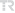## A note on Terai's conjecture concerning primitive Pythagorean triples

HACETTEPE JOURNAL OF MATHEMATICS AND STATISTICS, vol.50, no.4, pp.911-917, 2021 (SCI-Expanded)• Publication Type: Article / Article
• Volume: 50 Issue: 4
• Publication Date: 2021
• Doi Number: 10.15672/hujms.795889
• Journal Name:
• Journal Indexes: Science Citation Index Expanded (SCI-EXPANDED), Scopus, zbMATH, TR DİZİN (ULAKBİM)
• Page Numbers: pp.911-917
• Keywords: polynomial-exponential Diophantine equation, generalized Ramanujan-Nagell equation, primitive Pythagorean triple
• Bursa Uludag University Affiliated: Yes

#### Abstract

Let f,g be positive integers such that f > g, gcd(f,g) =1 and f not equivalent to g (mod 2). In 1993, N. Terai conjectured that the equation x(2) + (f(2) - g(2))(y) = (f(2) + g(2))(z) has only one positive integer solution (x, y, z) = (2 fg, 2, 2). This is a problem that has not been solved yet. In this paper, using elementary number theory methods with some known results on higher Diophantine equations, we prove that if f = 2(r)s and g = 1, where r, s are positive integers satisfying 2 inverted iota s, r >= 2 and s < 2(r-)(1), then Terai's conjecture is true.